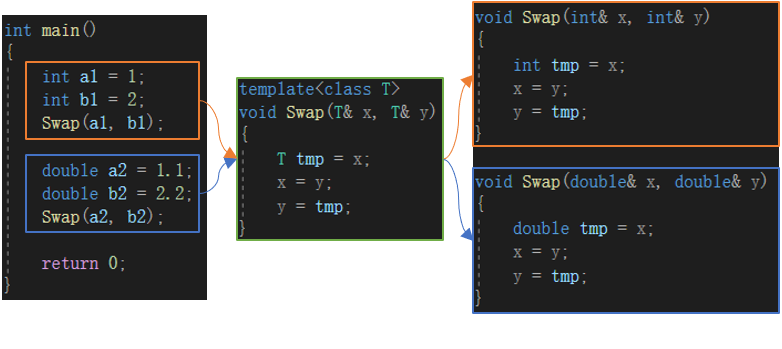WHCSRL 技术网

# C++初阶 —— 模板初阶

• 如重载函数仅为类型不同，代码复用率较低，新类型就要增加对应函数；
• 代码可维护性较低，一个出错可能所有重载均出错；

## 一，函数模板

函数模板代表了一个函数的家族，该函数模板与函数类型无关，在使用时被参数化，根据实参类型产生函数的特定类型版本；

### 函数模板格式

• template<typename T1，typename T2，...>
• 返回值类型 + 函数名 + (参数列表) { }

``template<class T>void Swap(T& x, T& y){	T tmp = x;	x = y;	y = tmp;}``

### 模板函数的原理

函数模板是一个蓝图，本身并不是函数，是编译器用使用方式产生特定具体类型的模具，所以其实模板就是将本来应该重复做的事交给了编译器；

在编译器编译的阶段，对于模板函数的使用，编译器需要根据传入的实参类型来推演生成对应类型的函数以供调用，即，当double类型使用模板函数时，编译器通过对实参类型的推演，将T确定为double，然后产生一份专门处理double类型的代码；注：内置类型也有构造函数，如 int a(1)；

• 用不同类型的参数使用函数模板时，称为函数模板的实例化；
• 模板参数实例化：隐式实例化和显示实例化；
• 隐式实例化，让编译器根据实参推演模板参数的实际类型；
• 显示实例化，在函数名后的<>中指定模板参数的实际类型；
``template<class T>void Swap(T x,T y){	T tmp = x;	x = y;	y = tmp;} int main(){	int a = 1;	double b = 2;    //显示实例化	Swap<int>(a, b); //或Swap(a, (int)b);	return 0;}``

``class A{public:	A(int a = 10)		:_a(a)	{}private:	int _a;};template<class T>T fun(int x){	T a(x);	return a;} int main(){    //只能显示实例化	A a = fun<A>(1);	return 0;}``

### 模板匹配原则

• 一个非模板函数可以和一个同名的函数模板同时存在，而且该函数模板还可以被实例化为这个非模板函数；
• 对于非模板函数和同名函数模板，如其他条件相同，在调动时会优先调用非模板函数而不会从该模板产生一个实例，如模板可以产生一个具有更好匹配的函数，则选择模板；
• 模板函数不允许自动类型转换，但普通函数可以进行自动类型转换；

• 有现成匹配的，直接调用，否则实例化模板生成；
• 需要转换的，优先选择实例化模板生成；
``template<class T>void Swap(T x,T y){	T tmp = x;	x = y;	y = tmp;} void Swap(int x, int y){	int tmp = x;	x = y;	y = tmp;} int main(){	int a = 1;	int b = 2;	Swap(a, b); //调用void Swap(int x, int y)	return 0;}``
``template<class T1, class T2>void Swap(T1 x,T2 y){	T1 tmp = x;	x = y;	y = tmp;} void Swap(int x, int y){	int tmp = x;	x = y;	y = tmp;} int main(){	Swap(1, 2); //使用void Swap(int x, int y)	Swap(1, 2.0); //使用void Swap(T1 x,T2 y)	return 0;}``

## 二，类模板

### 类模板格式

• template<class T1，class T2，...>
• class + 类模板名 { }
``template<class T>class stack{private:	T* _a; 	int _size;	int _capacity;};``

### 类模板实例化

• 类模板实例化和函数模板实例化不同，类模板实例化需在类模板名字后跟<实例化类型>，即显示实例化；
• 类模板名不是真正类，实例化后的结果才是真正的类；

• 普通类，类名即类型；
• 类模板，类型为类模板名<模板参数>，如stack<T>；
``template<class T>class stack{private:	T* _a;	int _size;	int _capacity;}; int main(){    //需显示实例化	stack<int>st1;	stack<double>st2;	return 0;}``
``template<class T>class stack{public:	stack(int capacity = 4)		:_a(new T[capacity])		,_top(0)		,_capacity(capacity)	{}	~stack()	{		delete[] _a;		_a = nullptr;		_top = _capacity = 0;	}	//类内声明，类外定义	void push(const T& x);private:	T* _a;	int _top;	int _capacity;};//类外定义，需加模板参数列表template<class T>void stack<T>::push(const T& x){	_a[_top] = x;	_top++;} int main(){	stack<int>st1;	stack<double>st2;	st1.push(1);	st2.push(1.1);	return 0;}``